# Civil Engineering - Engineering Economy

### Exercise :: Engineering Economy - Section 1

36.

The capital Recovery Factor (equal payments) of Capital Recovery Annuity is :

 A.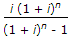B.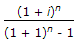C.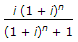D. None of these.

Explanation:

No answer description available for this question. Let us discuss.

37.

Current ratio is :

 A.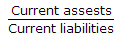B.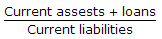C.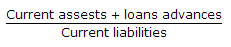D. None of these.

Explanation:

No answer description available for this question. Let us discuss.

38.

The interest calculated on the basis of 365 days a year, is known as :

 A. interest B. ordinary simple interest C. exact simple interest D. None of these

Explanation:

No answer description available for this question. Let us discuss.

39.

If S is the future capital accumulated in n years at the rate of interest i per annum, then present worth is :

 A.B. S(1 + i)n C. S(1 + i)1/n D. None of these.

Explanation:

No answer description available for this question. Let us discuss.

40.

In a cash-flow diagram :

 A. Time 0 is considered to be the present B. Time 1 is considered to be the end of time period 1 C. A vertical arrow pointing up indicates a positive cash flow D. An arrow pointing downward indicates a negative cash flow E. All of these